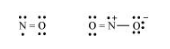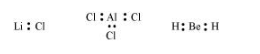# Define octet rule. Write its significance and limitations.

Question:

Define octet rule. Write its significance and limitations.

Solution:

The octet rule or the electronic theory of chemical bonding was developed by Kossel and Lewis. According to this rule, atoms can combine either by transfer of valence electrons from one atom to another or by sharing their valence electrons in order to attain the nearest noble gas configuration by having an octet in their valence shell.

$\ddot{o}:: c:: \ddot{0} \quad$ or $\quad \ddot{Q}=c=\ddot{0}$

The octet rule successfully explained the formation of chemical bonds depending upon the nature of the element.

Limitations of the octet theory:

The following are the limitations of the octet rule:

(a) The rule failed to predict the shape and relative stability of molecules.

(b) It is based upon the inert nature of noble gases. However, some noble gases like xenon and krypton form compounds such as $\mathrm{XeF}_{2}, \mathrm{KrF}_{2}$ etc.

(c) The octet rule cannot be applied to the elements in and beyond the third period of the periodic table. The elements present in these periods have more than eight valence electrons around the central atom. For example: $\mathrm{PF}_{5}, \mathrm{SF}_{0}$, etc(d) The octet rule is not satisfied for all atoms in a molecule having an odd number of electrons. For example, $\mathrm{NO}$ and $\mathrm{NO}_{2}$ do not satisfy the octet rule.(e) This rule cannot be applied to those compounds in which the number of electrons surrounding the central atom is less than eight. For example, LiCl, BeH $_{2}$, AlCl $_{3}$ etc. do not obey the octet rule.